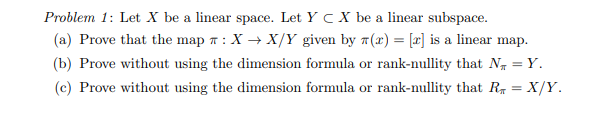Home / Answered Questions / Other / problem-1-let-x-be-a-linear-space-let-y-cx-be-a-linear-subspace-a-prove-that-the-map-x-x-y-given-by--aw941

# (Solved): Problem 1: Let X Be A Linear Space. Let Y CX Be A Linear Subspace. (a) Prove That The Map : X+X/Y Gi...Problem 1: Let X be a linear space. Let Y CX be a linear subspace. (a) Prove that the map : X+X/Y given by 7(x) = (2) is a linear map. (b) Prove without using the dimension formula or rank-nullity that N = Y. (c) Prove without using the dimension formula or rank-nullity that RX = X/Y.

We have an Answer from Expert